Chordal Graph

Cycle / Chord / Hole / Triangle

「環」是串成一圈的路線，沒有重複的點。

「弦」是環上不相鄰兩點之間的邊。

「洞」是一種特別的環，沒有弦。

「三角形」是三條邊的環，沒有弦。ICPC 7661

Chordal Graph（Monotone Transitive Graph）

「弦圖」。多於三條邊的環，必有弦。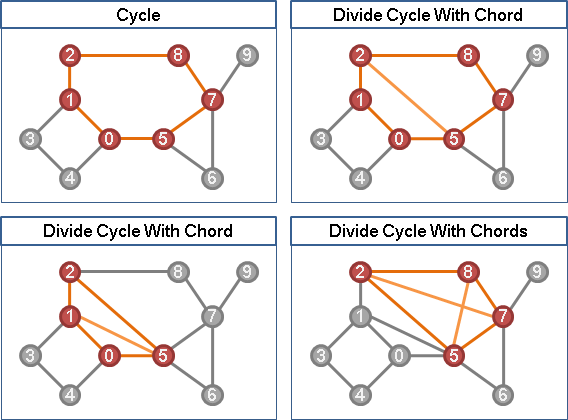Perfect Elimination Ordering

```「有向無環圖」擁有「拓樸順序」。反之亦然。
「弦圖」擁有「完美消除順序」。反之亦然。
```

Simplicial Vertex【註：古代名稱，今日看來詞不達意。】

「單體點」。該點的所有鄰居，之間必有邊。Perfect Elimination Ordering

「完美消除順序」。逐次刪除當下的任意一個單體點，所得到的順序。通常有許多種。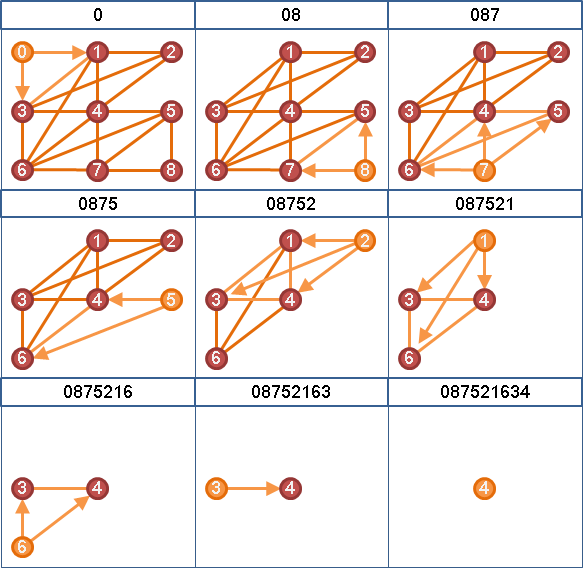Elimination Graph / Elimination Tree

「消除圖」。完美消除順序可以畫成有向圖。

「消除樹」。完美消除順序可以畫成有向森林。Perfect Elimination Ordering Testing

(⇒)弦圖必有單體點（容後證明）。弦圖隨便刪除一個點，仍是弦圖。弦圖可以遞迴刪除單體點。

(⇐)任意一個多於三條邊的環，此環之中，順序最早的點，稱之v。因為v的所有鄰居必有邊，所以此環必有弦。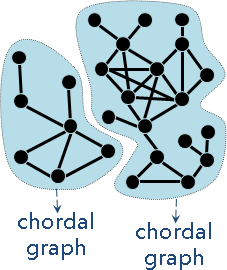S到AB都有邊，但是AB之間無邊，無法形成團。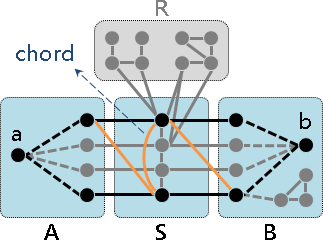A∪S要嘛是團、要嘛不是團。（a'∈A、b'∈S、a'∈A'、b'∈B'）

AB之間無邊，SB之間有邊，B將併入B'。

（a'∈A、b'∈A、a'∈A'、b'∈B'）

AB之間無邊，B不會併入A'B'。B跟A'B'S'皆不相交，那就只剩R'了。

B'至少佔據一點，(A'∪S')至少縮小一點。Chordal Graph Recognition

Graph Traversal

```有向無環圖的拓樸順序：特殊BFS順序、DFS逆序

```

Maximum Cardinality Search（MCS）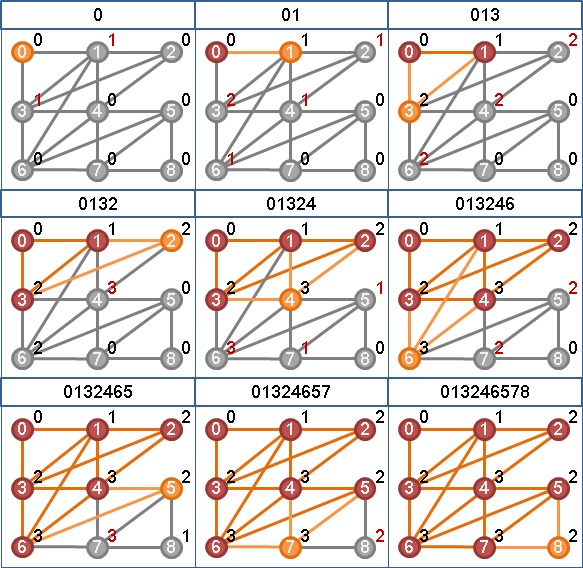BFS深入考慮細節。仔細安排小孩的拜訪順序：優先擴大團。

```i from V-1 to 0

```
```BFS：優先拜訪深度最小的點。
LexBFS：深度平手時，優先拜訪字串最大的點。
LexBFS-：字串平手時，優先拜訪索引值最小的點。
```Lexicographic Depth-first Search（LexDFS）

DFS深入考慮細節。仔細安排子孫的拜訪順序：優先擴大團。

```i from 0 to V-1

```
```DFS：優先拜訪深度最大的點。
LexDFS：深度平手時，優先拜訪字串最大的點。
LexDFS+：字串平手時，優先拜訪索引值最大的點。
```(⇒)遞迴刪除單體點：

(⇐)先前章節提過，完美消除順序必得弦圖。

MCS逆序、LexBFS逆序、LexDFS逆序，三者皆是如此。

Chordal Graph Recognition

MCS逆序、LexBFS逆序、LexDFS逆序，檢查是否為完美消除順序。挑一種檢查即可。

ICPC 2424 6308

```       GEN
/ | \
BFS MNS DFS
| / | \ |
LexBFS MCS LexDFS
```

MNS系統可以用來找到完美消除順序。

Interval Graph

Interval Graph

```(G == interval) iff (G == chordal && ~G == comparability)
if (G == interval) then (G == chordal)
if (G == interval) then (~G == comparability)
```

ICPC 2326

Circular-arc Graph

ICPC 4274

Split Graph

Split Graph

```(G == split) iff (G == chordal && ~G == chordal)
(G == split and G == permutation) iff (G == interval and ~G == interval)
```

「單調遞移圖Monotone Transitive Graph」就是擁有「完美消除順序」的圖。反之亦然？【尚待確認】

Chain Graph

Chain Graph

```(G == chain) iff (G == bipartite && C(G) == chordal)
C(G): make X and Y cliques
```

《Computing the Minimum Fill-In is NP-Complete》

Strongly Chordal Graph

Strongly Chordal Graph